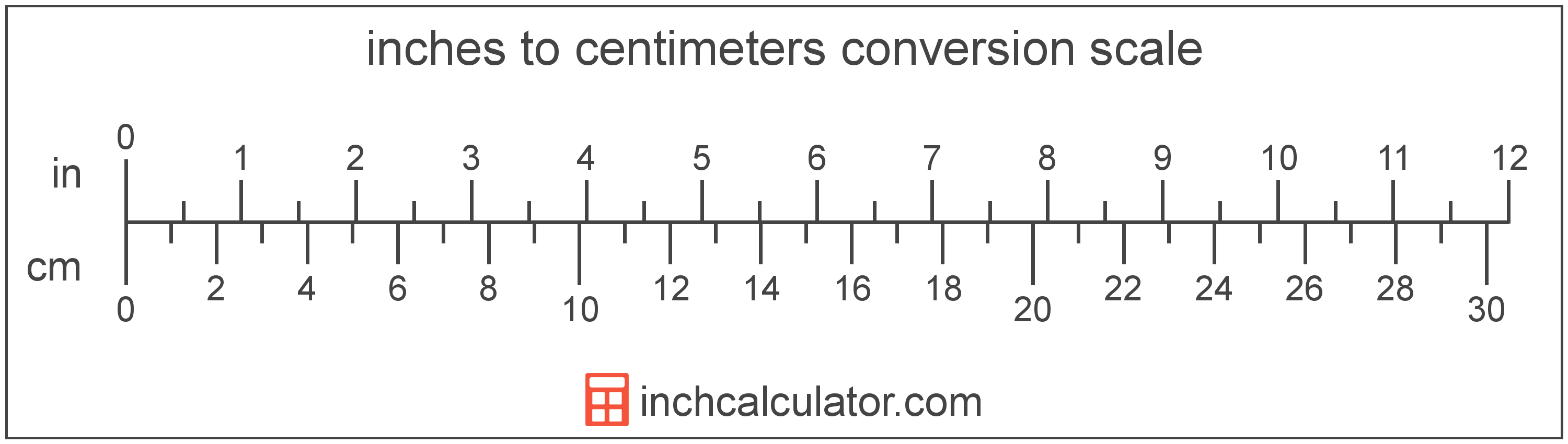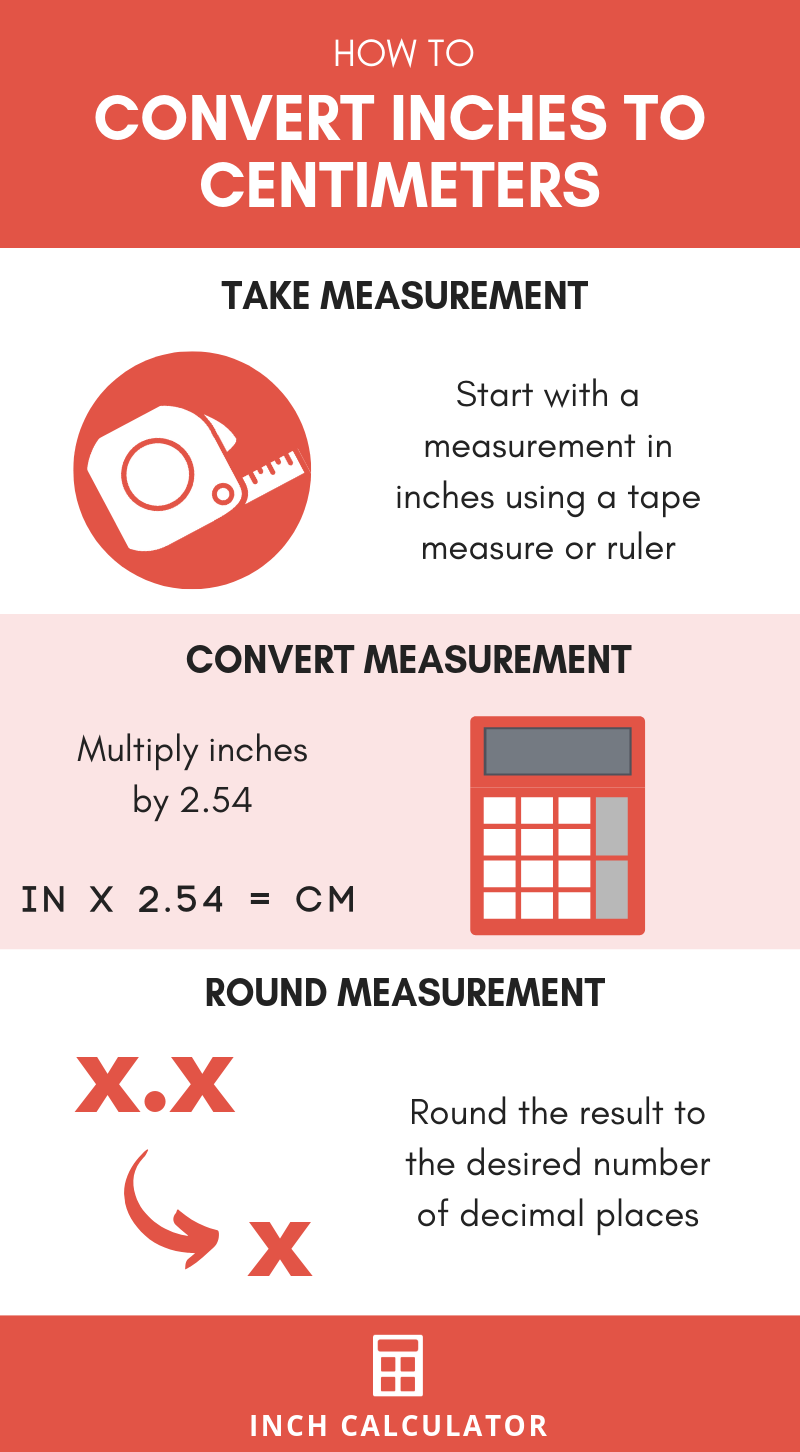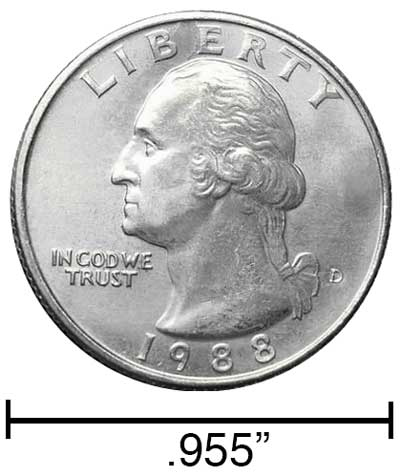# Inches to Centimeters Conversion Calculator

Enter the length below to convert inches to cm using decimal or fractional inches.

/
Results in Centimeters:1 in = 2.54 cm

Do you want to convert cm to inches?

## How to Convert Inches to Centimeters

To convert a measurement in inches to one in centimeters, you can use a simple formula.

Since one inch is equal to 2.54 centimeters, the formula used to convert inches to centimeters is:

centimeters = inches × 2.54

The length in centimeters is equal to the length in inches multiplied by 2.54.

For example, here's how to convert 5 inches to centimeters using the formula above.
centimeters = (5" × 2.54) = 12.7 cm### How Many Centimeters Are in an Inch?

There are 2.54 centimeters in an inch, which is why we use this value in the formula above.

1" = 2.54 cm

Our inch fraction calculator can add inches and centimeters together, and it also automatically converts the results to US customary, imperial, and SI metric values.## What is an Inch?

An inch is a unit of length equal to 1/12 of a foot or 1/36 of a yard. Because the international yard is legally defined to be equal to exactly 0.9144 meters, one inch is equal to 2.54 centimeters.

The inch is a US customary and imperial unit of length. Inches can be abbreviated as in; for example, 1 inch can be written as 1 in.

Inches can also be denoted using the symbol, otherwise known as a double-prime. Often a double-quote (") is used instead of a double-prime for convenience. A double-prime is commonly used to express 1 in as 1″.The standard ruler is 12 inches long and is a common tool for measuring a length in inches. Another frequently used tool to perform measurements in inches is a tape measure, which commonly comes in lengths from 6' - 35'. Other types of devices used to make measurements in inches include scales, calipers, measuring wheels, micrometers, yardsticks, and even lasers.

## What is a Centimeter?

One centimeter is equal to one-hundredth (1/100) of a meter, which is defined as the distance light travels in a vacuum in 1/299,792,458 of a second. One centimeter is equal to 0.393701 inches.

The centimeter, or centimetre, is a multiple of the meter, which is the SI base unit for length. In the metric system, "centi" is the prefix for hundredths, or 10-2. Centimeters can be abbreviated as cm; for example, 1 centimeter can be written as 1 cm.

Metric rulers typically have 30 cm, which are represented by 30 large tick marks. To get a rough idea of the actual length of a centimeter, a standard number 2 pencil is just about 1 cm thick.

We recommend using a ruler or tape measure for measuring length, which can be found at a local retailer or home center. Rulers are available in imperial, metric, or a combination of both values, so make sure you get the correct type for your needs.

Need a ruler? Try our free downloadable and printable rulers, which include both imperial and metric measurements.

## Inches to cm Conversion Chart

Inches to cm conversion chart showing equivalent length measurements in U.S. customary and metric.
Inches Centimeters
0.25 in 0.635 cm
0.5 in 1.27 cm
0.75 in 1.905 cm
1 in 2.54 cm
2 in 5.08 cm
3 in 7.62 cm
4 in 10.16 cm
5 in 12.7 cm
6 in 15.24 cm
7 in 17.78 cm
8 in 20.32 cm
9 in 22.86 cm
10 in 25.4 cm
11 in 27.94 cm
12 in 30.48 cm
13 in 33.02 cm
14 in 35.56 cm
15 in 38.1 cm
16 in 40.64 cm
17 in 43.18 cm
18 in 45.72 cm
19 in 48.26 cm
20 in 50.8 cm
25 in 63.5 cm
30 in 76.2 cm
35 in 88.9 cm
40 in 101.6 cm
45 in 114.3 cm
50 in 127 cm
55 in 139.7 cm
60 in 152.4 cm
65 in 165.1 cm
70 in 177.8 cm
75 in 190.5 cm
80 in 203.2 cm
85 in 215.9 cm
90 in 228.6 cm
95 in 241.3 cm
100 in 254 cm

## References

1. National Institute of Standards and Technology, Checking the Net Contents of Packaged Goods, Handbook 133 - 2019 Edition, https://nvlpubs.nist.gov/nistpubs/hb/2019/NIST.HB.133-2019.pdf